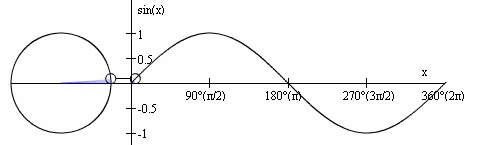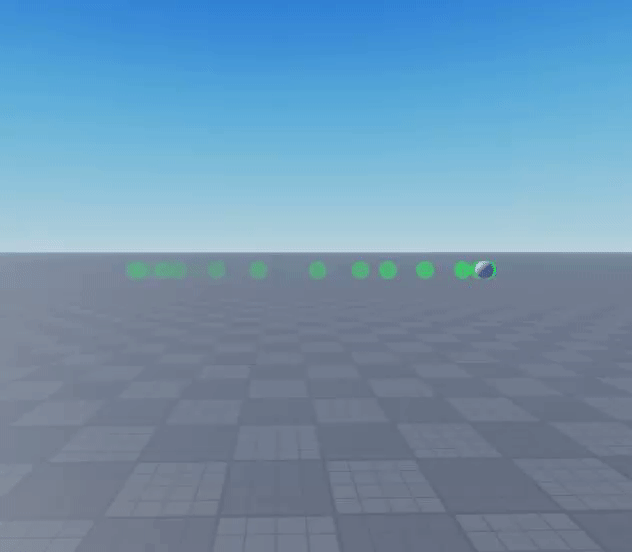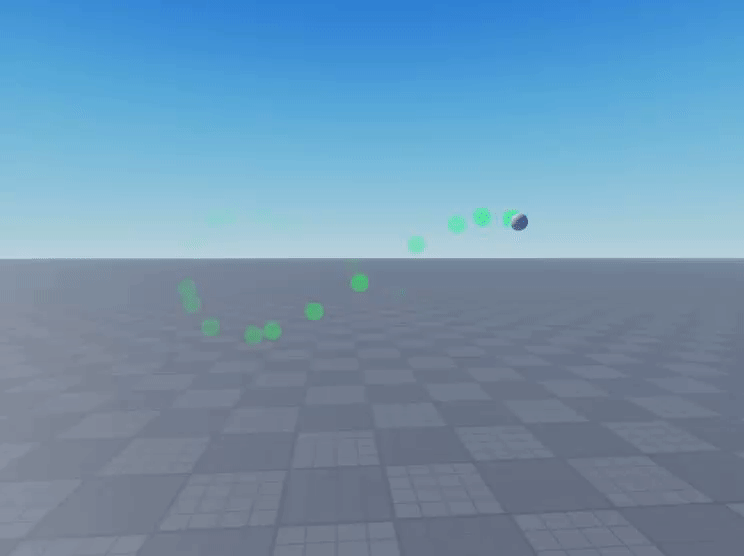# How can I make a part follow this path?

How can I make a part loop around in a infinite symbol?
CFrames or constraints, I need a way.

1 Like

You could maybe use math.sin() and math.cos().

I don’t know much about math, so I don’t know what sin or cos is.
Could you give me an example?

Back to back Cubic Beziers, Shameless plug How to make lerp Bezier Curves with RunService! [Chapter 1] but soon I might add a tutorial for cubics, but they aren’t really that complicated

1 Like

I tried looking at the tutorial, I don’t know how to make an infinite symbol out of that.

I feel honoured! When I get to my PC, I will make an update on the tutorial, and include your problem as an example

I’m not very good at explaining, so I can’t really get into detail about what sin and cosine is in math terminology. Here is a gif that might help understand:As you can see from the graph, sine is a number that moves back and forth from -1, to 1. You can use the wave-like motion in the sine function to sort of recreate the infinite symbol.

With this script, we can create the horizonal motion by applying the math.sin() function to the x-coordinate. In this example, I multiplied the current time with speed (5) to make it faster. I also multiplied the whole math.sin() function with strength (5) so that it made bigger movements.

``````local runservice = game:GetService('RunService')

local part = script.Parent
local x = part.Position.X
local y = part.Position.Y
local z = part.Position.Z
local speed = 5 -- feel free to change
local strength = 5 -- feel free to change

runservice.Heartbeat:Connect(function()
local currentTime = tick() -- current time is the elapsed
part.Position = Vector3.new(x + math.sin(currentTime*speed)*strength, y, z)
end)
``````Next, we can combine that with the vertical motion by applying the same math.sin() function to the Y-coordinate. I then doubled the speed and then made the strength lower to create the infinite symbol

``````local runservice = game:GetService('RunService')

local part = script.Parent
local x = part.Position.X
local y = part.Position.Y
local z = part.Position.Z
local speed = 5 -- feel free to change
local strength = 5 -- feel free to change

runservice.Heartbeat:Connect(function()
local currentTime = tick() -- current time is the elapsed
part.Position = Vector3.new(x + math.sin(currentTime*speed)*strength, y + math.sin(2*currentTime*speed)*strength/3 z)
end)
``````I hope this helps a little bit.

7 Likes

Well said! Nice to see appreciation of sine graphs!!

3 Likes

Thanks so much to you both, I’m going to use this for my view bobbing script.

1 Like